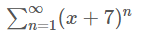# Find the values of x for which the series converges. (Enter your answer using interval notation.)

1.a. Find the values of x for which the series converges. (Enter your answer using interval notation.)
b. Find the sum of the series for those values of x.

2.a. Find the values of x for which the series converges. (Enter your answer using interval notation.)
b. Find the sum of the series for those values of x.